# 智能微网的国内外研究发展综述A Summary of Research and Development ofSmart Microgrid at Home and Abroad

• 全文下载: PDF(2403KB)    PP.70-87   DOI: 10.12677/SG.2019.92008
• 下载量: 429  浏览量: 673

With the increasing demand for renewable energy quality, it is difficult to meet the high-quality requirements of users by simply expanding the scale of the power grid. As an effective carrier, smart microgrid has been rapidly developed and paid attention. This paper first reviews the de-velopment of intelligent microgrid system, outlines the concept and foundation of intelligent mi-crogrid, and focuses on the structural system and modeling method of smart microgrid. The man-agement and allocation methods of multiple energy sources, and the stability of smart microgrid are analyzed. Finally, some problems existing in the smart microgrid system are described, and the development of the smart microgrid system is prospected and summarized.

1. 引言

1.1. 智能微网的简要发展史

1.2. 智能微网的基础结构

1) 电池是最常用的储能设备之一，主要有铅酸、镍镉、镍金属氢化物和锂离子电池。铅酸蓄电池适用于长时间储能，它的性能较差，使用周期短(1200~1800次)。镍镉电池与铅酸电池相比，镍镉电池具有更长的循环寿命、更高的能量密度和较低的维护保养，但成本过于昂贵。镍氢电池具有比镍镉电池高出25%~30%的能量密度，并与铅酸电池具有同等的循环寿命。与铅酸、镍镉和镍氢电池相比，锂电池的能量密度最高，但锂电池的投资成本和有限的寿命周期是锂电池的主要缺陷。为减轻光伏集成的负面影响，已经提出将电池存储系统集成到太阳能光伏系统中。

2) 飞轮储能装置具有寿命周期长、能量高、功率密度高的特点，但其缺点是有很高的摩擦损失，该装置可用来减少风能和太阳能系统产生的电力波动。

3) 超级电容器又称双层电容器，电容器与超级电容器的主要区别在于多孔膜的使用，多孔膜提供了两个电极之间的离子转移，因此电能可直接存储，响应时间非常小。此外，它的电容和能量密度值可以比电容器大几百到几千倍。与铅酸蓄电池相比，超级电容器具有较低的能量密度，但具有较高的功率密度、较长的循环寿命和约75%~80%的能源效率。但这项技术的主要缺点是成本过高，比铅酸电池高出5倍。超级电容器还可以缓解可再生能源的波动，这在文献  中也进行的详细的介绍。

4) 超导储能系统的生命周期非常长，从十个周期到数千个周期，效率高达95%，响应速度非常快，实现成本也很高，在功率因数、频率调节、暂态稳定性、电能质量等方面有良好的改善。利用与风电相结合的超导储能系统可以控制孤岛模式下微电网的频率和电压，当微电网在并网模式下运行时，超导储能系统可以提供恒定的功率流，克服风力发电引起的功率波动。

5) 钒氧化还原电池(VRB)是目前市面上的一种流动电池，与传统的储能电池(BESS)相比较具有寿命长、维护低、功率和能量独立、充放电响应快、效率高等优点，但与BESS相比运营和维护成本仍然相对较高。基于VRB的微电网文献比较有限，因为这项技术已经被商业化。

1) 满足并网模式下净有功/无功功率所需的负载/微源控制策略，及孤岛模式下电压、频率稳定性；

2) 提高关键型负载和敏感型负载的电能质量和可靠性；

3) 降低峰值以提高设备的评级；

4) 保持预期的操作和控制。

1.3. 智能微网的控制单元

2. 智能微网的建模及控制

2.1. 智能微网的建模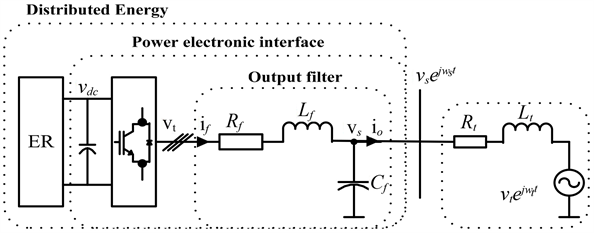Figure 1. Schematic diagram of distributed energy for electronic-interfaced

${L}_{g}\frac{\text{d}{i}_{o}}{\text{d}t}=-{R}_{g}{i}_{o}-j{\omega }_{s}{L}_{g}{i}_{o}-{v}_{g}{\text{e}}^{j\delta }+{v}_{s}$ (1)

$\frac{\text{d}\delta }{\text{d}t}={\omega }_{g}-{\omega }_{s}$ (2)

$\stackrel{˙}{x}=f\left(x\right)+g\left(x\right)u,\text{\hspace{0.17em}}\text{\hspace{0.17em}}y=h\left(x\right)$ (3)

$f\left(x\right)=\left[\begin{array}{c}-\frac{{R}_{g}}{{L}_{g}}{x}^{\left(1\right)}-\frac{{v}_{g}}{{L}_{g}}\mathrm{cos}{x}^{\left(3\right)}\\ -\frac{{R}_{g}}{{L}_{g}}{x}^{\left(2\right)}-\frac{{v}_{g}}{{L}_{g}}\mathrm{sin}{x}^{\left(3\right)}\\ {\omega }_{g}\end{array}\right]$ (4)

$g\left(x\right)=\left[\begin{array}{cc}\frac{1}{{L}_{g}}& {x}^{\left(2\right)}\\ 0& -{x}^{\left(1\right)}\\ 0& -1\end{array}\right],\text{\hspace{0.17em}}\text{\hspace{0.17em}}h\left(x\right)=\left[\begin{array}{c}{x}^{\left(1\right)}\\ {x}^{\left(2\right)}\end{array}\right]$ (5)

$\stackrel{˙}{x}={A}_{0}x+{B}_{0}u+ϵ,\text{\hspace{0.17em}}\text{\hspace{0.17em}}y={C}_{0}x$ (6)

 (7)

${B}_{0}=g\left({x}_{0}\right),\text{\hspace{0.17em}}{C}_{0}=\left[\begin{array}{cc}{I}_{2×2}& {O}_{2×1}\end{array}\right]$ (8)

${A}_{0}=\left[\begin{array}{ccc}\frac{{R}_{g}}{{L}_{g}}& {\omega }_{b}& 0\\ -{\omega }_{b}& -\frac{{R}_{g}}{{L}_{g}}& -\frac{{\nu }_{b}}{{L}_{g}}\\ 0& 0& 0\end{array}\right],\text{\hspace{0.17em}}\text{\hspace{0.17em}}{B}_{0}=\left[\begin{array}{cc}\frac{1}{{L}_{g}}& 0\\ 0& 0\\ 0& -1\end{array}\right]$ (9)

$G\left(s\right)={C}_{0}{\left(sI-{A}_{0}\right)}^{-1}{B}_{0}$ (10)

$G\left(s\right)=\frac{1}{{L}_{g}{A}_{ol}\left(s\right)}\left[\begin{array}{cc}s+\frac{{R}_{g}}{{L}_{g}}& \frac{{\nu }_{b}{\omega }_{b}}{s}\\ -{\omega }_{b}& \frac{{v}_{b}}{s}\left(s+\frac{{R}_{g}}{{L}_{g}}\right)\end{array}\right]$ (11)

${A}_{ol}\left(s\right)={s}^{2}+2\frac{{R}_{g}}{{L}_{g}}s+{\left(\frac{{R}_{g}}{{L}_{g}}\right)}^{2}+{\omega }_{b}^{2}$ (12)

${x}_{k+1}={A}_{d}{x}_{k}+{B}_{d}{u}_{k},\text{\hspace{0.17em}}{y}_{k}={C}_{d}{x}_{k}$ (13)

${A}_{d}={\text{e}}^{{T}_{s}{A}_{0}},\text{\hspace{0.17em}}\text{\hspace{0.17em}}{B}_{d}={\int }_{0}^{{T}_{s}}{\text{e}}^{{A}_{0}\eta }{B}_{0}\text{d}\eta ,\text{\hspace{0.17em}}\text{\hspace{0.17em}}{C}_{d}={C}_{0}$ (14)

2.2. 智能微网的控制

2.2.1. 智能微网的分层控制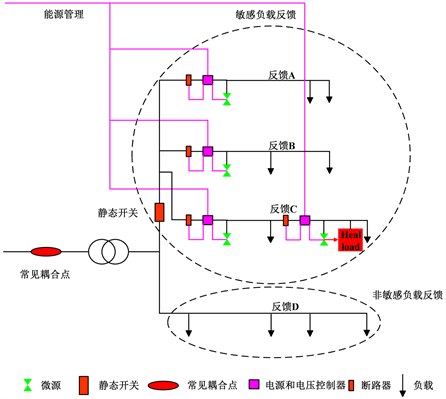Figure 2. Smart microgrid architecture

1) 由微控制器(MCS)和负载控制器(LCS)组成的局部控制器；

2) 微电网中央控制器(MGCC)；

3) 分销管理系统(DMS)。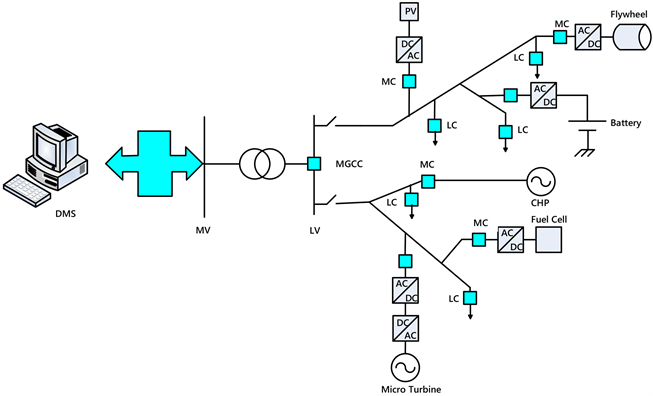Figure 3. Hierarchical control of smart microgrid

2.2.2. 智能微网的微源控制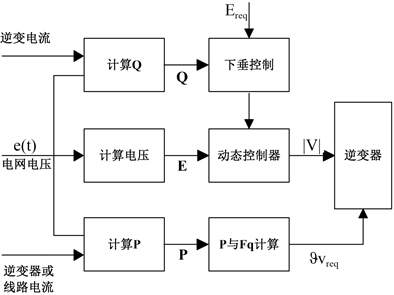Figure 4. Microsource controller

3. 智能微网的能源管理及稳定性

3.1. 可再生能源

3.2. 能源管理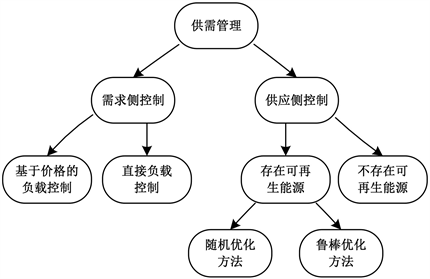Figure 5. Microgrid supply and demand management

3.3. 智能微网的稳定性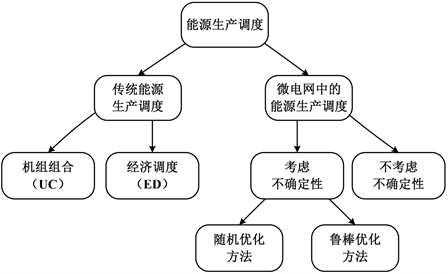Figure 6. Microgrid energy production scheduling

1) 智能微电网可在并网模式和孤岛模式下运行。并网模式下，微电网的功率流是双向的。孤岛模式下，微电网的供电必须满足负载的需求。

2) 智能微电网的结构多样化。由于分布式发电机(DG)的种类繁多，不同DG可以构成各种微电网结构，其运行模式多样化导致了微电网稳定问题多样化的特点。

3) DG控制策略对微电网电压和频率的调节有显著影响，不同控制策略下的电压和频率调节特性差异很大。

4) 输出阻抗小，过流能力小。与同步发电机相比，逆变器接口的输出阻抗和过流能力非常小，因此这些DG的响应时间比同步发电机更快，导致微电网受到大扰动时的操作过程发生改变，是微电网的瞬态分析的重要特性之一。

5) 智能微电网的储能。直流电容器中存储的能量较小，当发生大的干扰时，DG用来满足其不同的能量需求，使得储能系统保持稳定。

3.3.1. 并网微电网稳定性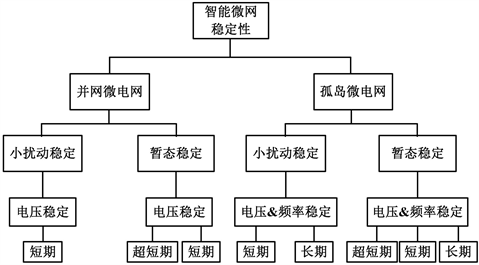Figure 7. Microgrid stability classification

3.3.2. 孤岛微电网稳定性

4. 展望

4.1. 能源优化问题

4.2. 供需匹配问题

4.3. 成本优化问题

4.4. 保护问题

5. 结论